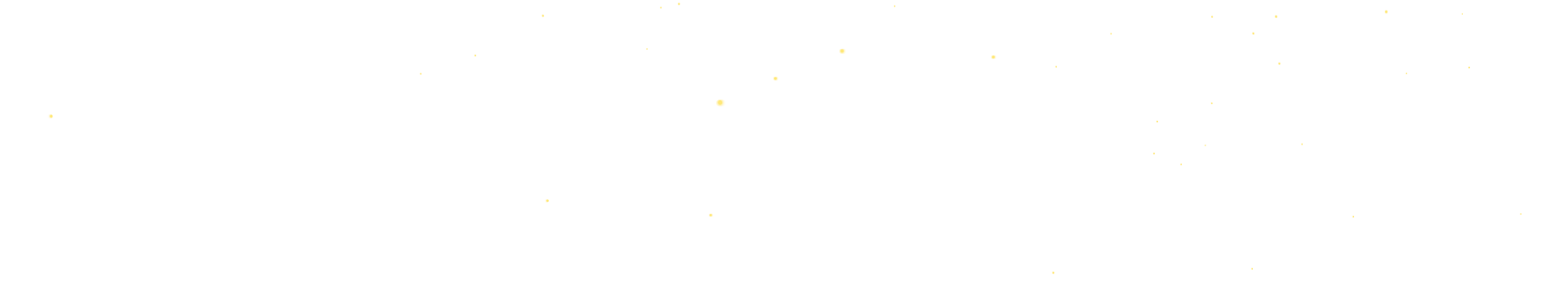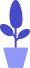# Detailed Implementation of Python Pygame Snake Game (Part 2)

Estimated reading: 11 minutes 58 views

In this section, we will introduce other custom functions used in the runGame() function mentioned in the previous section.

## drawFood() function

The drawFood() function is used to draw food. The parameters it accepts are the pygame.Surface object of the window and the dictionary object representing the coordinates.

The code for the drawFood() function is shown below.

``````def drawFood(screen, food):
x = food["x"] * cellSize
y = food["y"] * cellSize
pygame.draw.rect(screen, YELLOW, (x, y, cellSize, cellSize))``````

The result of multiplying the value corresponding to the key “x” of the dictionary food by the variable cellSize is assigned to the variable x, and the result of multiplying the value corresponding to the key “y” of the dictionary food by the variable cellSize is assigned to the variable y. Because the coordinates of food are relative to the coordinates on the map, not the coordinates of the real window, the corresponding pixel position on the window can only be obtained after multiplying by cellSize.

Then, call the pygame.draw.rect() function to draw a small square filled with yellow.

## drawSnake() function

The drawSnake() function is used to draw the snake. The parameters it accepts are the pygame.Surface object of the window and the list representing the snake.

The code for the drawSnake() function is shown below.

``````def drawSnake(screen, snakeCoords):
for coord in snakeCoords:
x = coord["x"] * cellSize
y = coord["y"] * cellSize
pygame.draw.rect(screen, DARKGREEN, (x, y, cellSize, cellSize))
pygame.draw.rect(screen, GREEN,(x + 4, y + 4, cellSize - 8, cellSize - 8))``````

Use a for loop to iterate over all the elements in the snakeCoords list, assigning each element to the variable coord.

In each loop body, multiply the value corresponding to the key “x” of the dictionary coord by the variable cellSize, then assign the result to the variable x, multiply the value corresponding to the key “y” of the dictionary coord by the variable cellSize, and then put the result Assign it to the variable y. The variables x and y correspond to the pixel positions on the window.

Then call the pygame.draw.rect() function to draw a small square filled with dark green, and call the pygame.draw.rect() function again to draw a small light green square in the dark green square. A large square and a small square together form a section of the snake’s body.

## drawScore() function

The drawScore() function is used to draw the score. The parameters it accepts are the pygame.Surface object of the window and the variable representing the score.

The code for the drawScore() function is shown below.

``````def drawScore(screen,score):
font = pygame.font.SysFont("SimHei", 30)
scoreSurf = font.render("得分: " + str(scoer), True, WHITE)
scoreRect = scoreSurf.get_rect()
scoreRect.topleft = (windowsWidth - 200, 50)
screen.blit(scoreSurf, scoreRect)``````

Call the pygame.font.SysFont() function, draw the string “score:” and the value of the variable score to the interface, draw in anti-aliasing mode, the text color is white, and assign the generated Font object to the variable scoreSurf.

Then get the rectangle object of scoreSurf and assign it to the variable scoreRect. Specify the coordinates of the upper left corner of the scoreRect as (windowsWidth – 200, 50). Then, call the blit() function to copy the scoreSurf object to the screen Surface.

## moveSnake() function

The moveSnake() function is used to move the snake. The parameters it accepts are a variable representing the direction and a list representing the snake. The moveSnake() function will add a snake head element to the list according to the direction.

The code for the moveSnake() function is shown below.

``````def moveSnake(direction, snakeCoords):
if direction == UP:
newHead = {"x": snakeCoords[HEAD][ "x"], "y": snakeCoords[HEAD][ "y"] - 1}
elif direction == DOWN:
newHead = {"x": snakeCoords[HEAD][ "x"], "y": snakeCoords[HEAD][ "y"] + 1}
elif direction == LEFT:
newHead = {"x": snakeCoords[HEAD][ "x"] - 1, "y": snakeCoords[HEAD][ "y"]}
elif direction == RIGHT:
newHead = {"x": snakeCoords[HEAD][ "x"] + 1, "y": snakeCoords[HEAD][ "y"]}

If the variable direction is equal to UP, indicating that the direction of the snake is upward, then create a new snake head element, the value of the “x” key is the same as the value of the original “x” key of the snake head, and the value of the “y” key is the original The value of the “y” key of the snake head minus 1 unit.

Otherwise, if the variable direction is equal to DOWN, indicating that the direction of the snake is downward, then create a new snake head element, the value of the “x” key is the same as the value of the “x” key of the original snake head, and the value of the “y” key is unchanged. The value is the value of the “y” key of the original snake head plus 1 unit.

Otherwise, if the variable direction is equal to LEFT, indicating that the direction of the snake is to the left, then create a new snake head element, the value of the “x” key is the value of the “x” key of the original snake head minus 1 unit, “y” “The value of the key is the same as the value of the “y” key of the original snake head.

Otherwise, if the variable direction is equal to RIGHT, indicating that the direction of the snake is to the right, then create a new snake head element, the value of the “x” key is the value of the “x” key of the original snake head plus 1 unit, “y” “The value of the key is the same as the value of the “y” key of the original snake head.

Then, insert this newly created dictionary element into the first position of the snake list.

## isEattingFood() function

The isEattingFood() function is used to determine whether the snake has eaten food. The parameters it accepts are a list representing the snake and a variable representing the location of the food.

The code for the isEattingFood() function is shown below.

``````def isEattingFood(snakeCoords, food):
if snakeCoords[HEAD]["x"] == food["x"] and snakeCoords[HEAD]["y"] == food["y"]:
food["x"] = random.randint(0, mapWidth - 1)
food["y"] = random.randint(0, mapHeight - 1)
else:
del snakeCoords[-1]
``````

First determine whether the value of the “x” key and “y” key of the first element of the list snakeCoords is equal to the value of the “x” key and “y” key of the variable food. We introduced earlier that the variable HEAD is equal to 0.

If they are equal, it means that the snake head touched the food. Then reset the value of the “x” key and “y” key of the variable food. Note that for lists or dictionaries, modifying the content of the parameter inside the function will affect the object outside the function.

If not equal, remove the last element in the snakeCoords list. When I introduced the moveSnake() function, I mentioned that moving the snake is actually adding a new element. For example, it starts with 3 elements, and moves one step to the right, it becomes 4 elements. If no food is eaten at this time, in order to keep the number of elements unchanged, the last element must be deleted, so as to ensure that the number of elements in the snakeCoords list has not changed, and is still 3 elements.

## isAlive() function

The isAive() function is used to determine whether the snake is dead. The parameter it accepts is a list of snakes.

The code for the isAive() function is shown below.

``````def isAlive(snakeCoords):
tag = True
if snakeCoords[HEAD]["x"] == -1 or snakeCoords[HEAD]["x"] == mapWidth or snakeCoords[HEAD]["y"] == -1 or snakeCoords[HEAD]["y"] == mapHeight:
tag = False        #Snake hits a wall
for snake_body in snakeCoords[1:]:
if snake_body["x"] == snakeCoords[HEAD]["x"] and snake_body["y"] == snakeCoords[HEAD]["y"]:
tag = False   # The greedy snake touches its own body
return tag``````

First, set the variable tag to True. Then, determine whether the x-coordinate of the snake head on the map is equal to −1, or whether the x-coordinate of the snake’s head is equal to mapWidth, or whether the y-coordinate of the snake’s head is equal to −1, or whether the y-coordinate of the snake’s head is equal to mapHeight, as long as any one of them is satisfied condition, it means that the snake head touched the wall, then the variable tag is set to False.

Then use a for loop to traverse the second and subsequent elements in the snakeCoords list, assigning each element to the variable snake_body, which represents the snake’s body.

In the loop body, judge whether the values ​​corresponding to the keys “x” and “y” of the snake_body in the dictionary are equal to the first element of the snakeCoords list, that is, the values ​​corresponding to the keys “x” and “y” of the snake head. To the snake’s body, then set the variable tag to False.

Finally, the function returns the variable tag. If tag is True, the snake is alive; if tag is False, the snake is dead.

## gameOver() function

The gameOver() function controls the end of the entire program, and the parameter it accepts is the pygame.Surface object of the window.

The code for the gameOver() function is shown below.

``````def gameOver(screen):
#Load game over image
screen.fill(WHITE)
screen.blit(gameOver, (0, 0))
#Load game over message
font = pygame.font.SysFont("SimHei", 36)
tip = font.render("Press Q or ESC to exit the game, press other keys to restart the game", True, (65, 105, 225))
screen.blit(tip, (30, 500))
#Display the content on the Surface object
pygame.display.update()
while True:
for event in pygame.event.get():
if event.type == pygame.QUIT:
terminate()
elif event.type == pygame.KEYDOWN:
if event.key == pygame.K_ESCAPE or event.key == pygame.K_q:
terminate()
else:
return #End this function, restart the game``````

First call the screen.fill() function to fill the window with white.

Then call the image() function to load the “gameover.png” image in the Pygame window and create a Surface object called gameOver. Then we call the blit() function to copy the gameOver object to the screen Surface. Copies the gameOver to the specified upper-left position (0, 0) via blit().

Then call the pygame.font.SysFont function, and draw the string “Press Q or ESC to exit the game, and press other keys to restart the game” on the interface. Then we call the blit() function to copy the tip object to the screen Surface.

Call the pygame .display.update() function to display everything drawn on the Surface object to the window.

Next, use a while loop to listen for keyboard events. In the event loop, determine the event type, if it is QUIT, then call the terminate function to terminate the program. Otherwise, if the event type is KEYDOWN, then the event object will have a key property to identify which key was pressed.

If the key attribute is equal to K_ESCAPE or K_q, it means that the user pressed the Esc or Q key, which means that the player wants to end the program, then the processing method is the same as clicking to close the window, and the terminate() function is called to terminate the program. Otherwise, it means that the user pressed another key, end this function, and restart the game.

When this function is executed, the game interface shown in Figure 1 will appear. At this time, you can press the Q key and the Esc key to end the program, or you can press any key to restart a game.figure 1

## terminate() function

The terminate() function terminates the program. Let’s take a look at the code for this function.

``````def terminate():
pygame.quit()
sys.exit()``````

Call the pygame.quit() function, which is a function corresponding to init(). It needs to be called before exiting the program. Then it will exit Pygame. Call the sys.exit() function to exit the main program.

## Call the entry function

Finally, we only need to call the entry function main(), and the program can start running.

``main()``

At this point, our snake game is complete. This is a complete game in the true sense, with a start interface and an end interface, a game background, and background music. Try to play and then go back and look at the code of the game, it will be more helpful to understand the code.